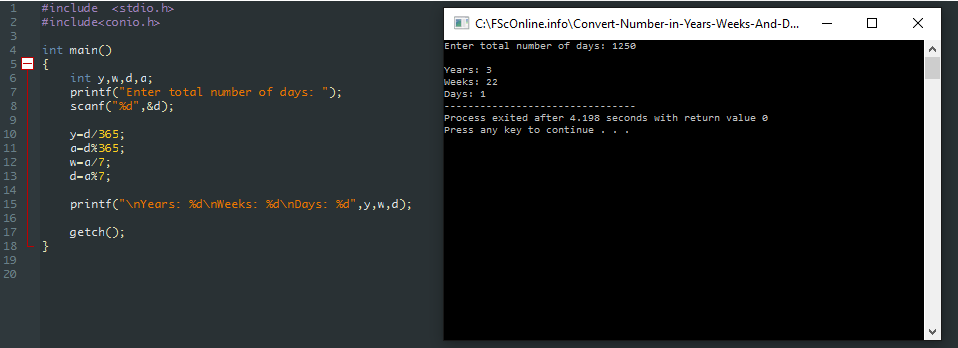Program to convert given number of days into years, weeks and days

1. Get number of days from user
2. Divide number of days with 365 to get number of years
3. For week take mod with 365 days and divide it with 7 you’ll get weeks
4. And for days take mod with 365 and then take mode with 7 you’ll get days

### C Code

Related Posts
```#include <stdio.h>
#include<conio.h>

int main()
{
int y,w,d,a;
clrscr();
printf("Enter total number of days:");
scanf("%d",&d);

y=d/365;
a=d%365;
w=a/7;
d=a%7;

printf("\nYears: %d\nWeeks: %d\nDays: %d",y,w,d);

getch();
}
```

### C++ Code

```#include <iostream>
#include <conio.h>

using namespace std;

int main()
{
int y,w,d,a;
cout << "Enter total number of days: ";
cin >> d;

y=d/365;
a=d%365;
w=a/7;
d=a%7;

cout << endl << "Years: "<< y
<< endl << "Weeks: " << w
<< endl << "Days: " << d;

return 0;
}
```

#### Output:#### Other Related Search Terms

1. C Program to convert given number of days into years, weeks and days
2. C++ program to convert given number of days into years, weeks and days
3. C and C++ program for check remaining years, weeks and days by giving number of days.
You might also like
1 Comment
1. Avneet Kaur says

you forgot to mention leap year.

This website uses cookies to improve your experience. We'll assume you're ok with this, but you can opt-out if you wish. Accept Read More